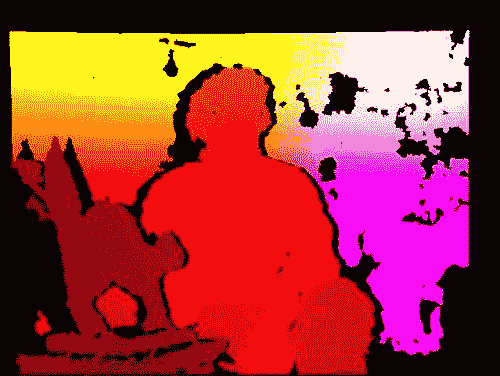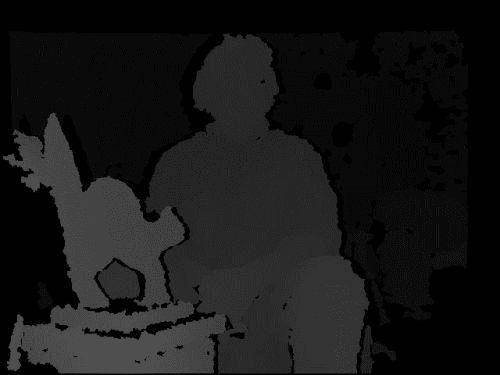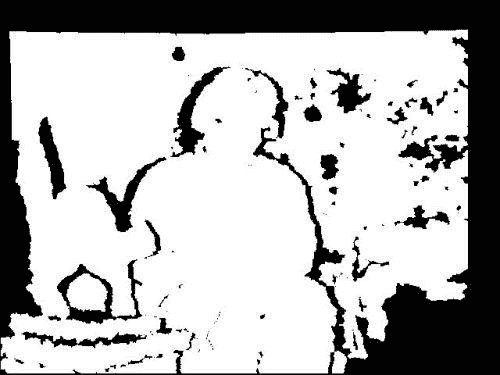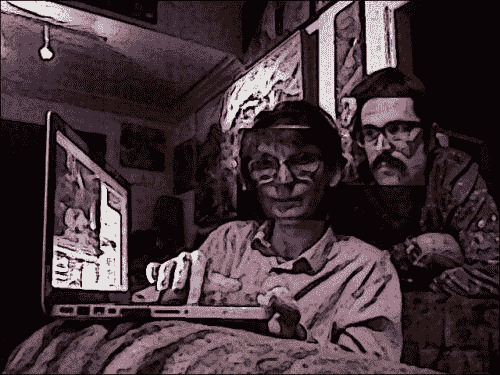# 创建模块

import numpy


import numpy
import utils


import depth


# 从深度相机捕获帧

# Devices.
CV_CAP_OPENNI = 900 # OpenNI (for Microsoft Kinect)
CV_CAP_OPENNI_ASUS = 910 # OpenNI (for Asus Xtion)
# Channels of an OpenNI-compatible depth generator.
CV_CAP_OPENNI_DEPTH_MAP = 0 # Depth values in mm (CV_16UC1)
CV_CAP_OPENNI_POINT_CLOUD_MAP = 1 # XYZ in meters (CV_32FC3)
CV_CAP_OPENNI_DISPARITY_MAP = 2 # Disparity in pixels (CV_8UC1)
CV_CAP_OPENNI_DISPARITY_MAP_32F = 3 # Disparity in pixels (CV_32FC1)
# Channels of an OpenNI-compatible RGB image generator.
CV_CAP_OPENNI_BGR_IMAGE = 5
CV_CAP_OPENNI_GRAY_IMAGE = 6


• 深度图是灰度图像，其中每个像素值是从相机到表面的估计距离。 具体而言，来自CV_CAP_OPENNI_DEPTH_MAP通道的图像给出的距离为浮点数毫米。
• 点云图是彩色图像，其中每种颜色对应于一个空间尺寸（xyz）。 具体而言，从相机的角度来看，CV_CAP_OPENNI_POINT_CLOUD_MAP通道会生成 BGR 图像，其中 B 为x（蓝色为右），G 为y（绿色为上），R 为z（红色为深）。 值以米为单位。
• 视差图是灰度图像，其中每个像素值是表面的立体视差。 为了概念化立体视差，我们假设我们叠加了一个场景的两个图像，这些图像是从不同的角度拍摄的。 结果就像看到双重图像。 对于场景中任何一对孪生对象上的点，我们都可以以像素为单位测量距离。 该测量是立体差异。 与远处的物体相比，附近的物体表现出更大的立体视差。 因此，附近的物体在视差图中显得更亮。
• 有效深度掩码显示给定像素处的深度信息是有效的（由非零值表示）还是无效的（由零值表示）。 例如，如果深度相机依靠红外照明器（红外闪光灯），则深度信息在被该光遮挡（阴影）的区域中无效。# 从视差图创建遮罩

def createMedianMask(disparityMap, validDepthMask, rect = None):
if rect is not None:
x, y, w, h = rect
disparityMap = disparityMap[y:y+h, x:x+w]
median = numpy.median(disparityMap)
return numpy.where((validDepthMask == 0) | \
(abs(disparityMap - median) < 12),
1.0, 0.0)


# 带遮罩的复制操作

def copyRect(src, dst, srcRect, dstRect, mask = None,
interpolation = cv2.INTER_LINEAR):
"""Copy part of the source to part of the destination."""

x0, y0, w0, h0 = srcRect
x1, y1, w1, h1 = dstRect

# Resize the contents of the source sub-rectangle.
# Put the result in the destination sub-rectangle.
dst[y1:y1+h1, x1:x1+w1] = \
cv2.resize(src[y0:y0+h0, x0:x0+w0], (w1, h1),
interpolation = interpolation)
else:
if not utils.isGray(src):
# Convert the mask to 3 channels, like the image.
# Perform the copy, with the mask applied.
dst[y1:y1+h1, x1:x1+w1] = \
interpolation = \
cv2.INTER_NEAREST),
cv2.resize(src[y0:y0+h0, x0:x0+w0], (w1, h1),
interpolation = interpolation),
dst[y1:y1+h1, x1:x1+w1])


def swapRects(src, dst, rects, masks = None,
interpolation = cv2.INTER_LINEAR):
"""Copy the source with two or more sub-rectangles swapped."""

if dst is not src:
dst[:] = src

numRects = len(rects)
if numRects < 2:
return

# Copy the contents of the last rectangle into temporary storage.
x, y, w, h = rects[numRects - 1]
temp = src[y:y+h, x:x+w].copy()

# Copy the contents of each rectangle into the next.
i = numRects - 2
while i >= 0:
interpolation)
i -= 1

# Copy the temporarily stored content into the first rectangle.
copyRect(temp, dst, (0, 0, w, h), rects, masks[numRects - 1],
interpolation)


# 修改应用

class CameoDepth(Cameo):
def __init__(self):
self._windowManager = WindowManager('Cameo',
self.onKeypress)
device = depth.CV_CAP_OPENNI # uncomment for Microsoft Kinect
#device = depth.CV_CAP_OPENNI_ASUS # uncomment for Asus Xtion
self._captureManager = CaptureManager(
cv2.VideoCapture(device), self._windowManager, True)
self._faceTracker = FaceTracker()
self._shouldDrawDebugRects = False
self._curveFilter = filters.BGRPortraCurveFilter()
def run(self):
"""Run the main loop."""
self._windowManager.createWindow()
while self._windowManager.isWindowCreated:
self._captureManager.enterFrame()
self._captureManager.channel = \
depth.CV_CAP_OPENNI_DISPARITY_MAP
disparityMap = self._captureManager.frame
self._captureManager.channel = \
self._captureManager.channel = \
depth.CV_CAP_OPENNI_BGR_IMAGE
frame = self._captureManager.frame
self._faceTracker.update(frame)
faces = self._faceTracker.faces
for face in faces
]
rects.swapRects(frame, frame,
[face.faceRect for face in faces], masks)
filters.strokeEdges(frame, frame)
self._curveFilter.apply(frame, frame)
if self._shouldDrawDebugRects:
self._faceTracker.drawDebugRects(frame)
self._captureManager.exitFrame()
self._windowManager.processEvents()


if __name__=="__main__":
#Cameo().run() # uncomment for single camera
#CameoDouble().run() # uncomment for double camera
CameoDepth().run() # uncomment for depth camera# 总结

• 《NumPy Cookbook》，伊万·伊德里斯
• 《OpenCV 2 计算机视觉应用设计手册》，RobertLaganière，该手册在台式机上使用 OpenCV 的 C++ API
• 《通过实用的计算机视觉项目掌握 OpenCV》，由多位作者撰写，其将 OpenCV 的 C++ API 用于多个平台
• 即将出版的书《OpenCV for iOS How-to》，使用针对 iPhone 和 iPad 的 OpenCV 的 C++ API
• 我即将出版的《OpenCV Android 应用编程》，使用了用于 Android 的 OpenCV 的 Java API